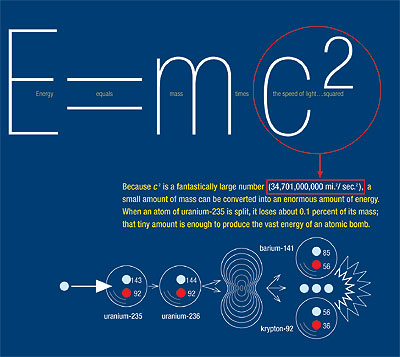A# mass-energy relationshipThe mass-energy relationship is the famous equation, discovered by Einstein: E = mc2, where E is energy, m is the equivalent amount of mass, and c is the velocity of light. This relation shows that, effectively, mass and energy are two sides of the same coin. It is also the basis for understanding the principle of nuclear fusion.